The Triskele Curve

loopspace

2nd August 2018

# 1 Introduction

I recently came across Triskele Globes. It was sufficiently near to Christmas that I thought I'd have a go at making one as they look quite festive. I was right: they do look quite festive. However, looking very closely at them then I wasn't quite sure that the fold was along the right curve. There seemed to be a couple of points of deformation, particularly at the corners. So I thought I'd have a look at figuring out what the curve ought to be.

# 2 Defining the Curve in 3D

Let's generalise. Suppose we wrinkle a piece of paper along its length so that its cross section is the same all through.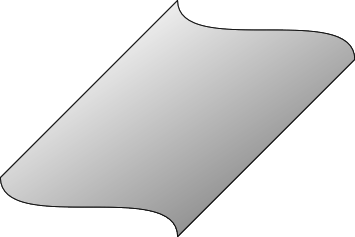Let's assume that we start with a piece of paper lying in the positive quadrant of the $x-y$–plane up against the axes. We then wrinkle it in the $y$–direction, meaning that as you travel in the $y$–direction then the paper changes height but along the $x$–direction then it stays constant. We keep it above the positive quadrant and so that its edges stay above the axes (though we give ourselves leave to move it vertically).

This means that the paper is described by a parametrisation of the form $\left(s,f\left(t\right),g\left(t\right)\right)$.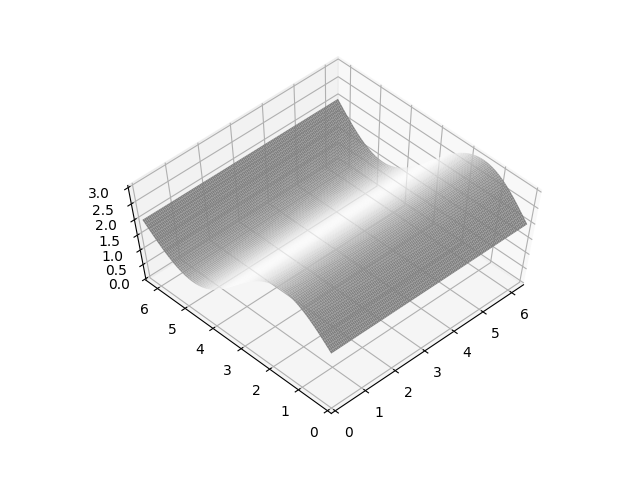We want to fold it straight down so that the folded part has constant cross section in the $z$–direction. Once it has been folded, the bottom edge of the paper will be contained in a horizontal plane since we can only flex the paper in one direction at a time. We can therefore arrange it so that the bottom edge (once folded) lies in the $x-y$–plane.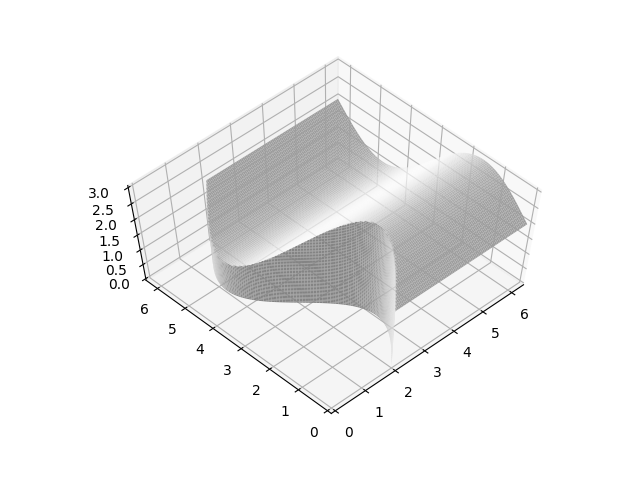Pre-folding, this edge was in the $y-z$–plane and post-folding it is in the $x-y$–plane. Therefore the distance along the paper from the fold to the $y-z$–plane prior to folding is the same as the distance along the paper from the fold to the $x-y$–plane after folding. That is to say, the fold lies on the plane $x=z$.

On the paper, that translates to $s=g\left(t\right)$. Thus the fold curve is $\left(g\left(t\right),f\left(t\right),g\left(t\right)\right)$.

After folding, this then continues down to the $x-y$–plane and the parametrisation of the folded part is therefore $\left(g\left(t\right),f\left(t\right),s\right)$. A full parametrisation of the paper is therefore:

 $\left(\mathrm{max}\left(s,g\left(t\right)\right),f\left(t\right),\mathrm{min}\left(s,g\left(t\right)\right)\right)$

# 3 Defining the Curve in 2D

The previous section defines the curve in space, but what we want to do is to draw it on the paper at the outset. To do that, we need to draw it on the paper and then "unwrinkle" the paper. If we let $\left(u,v\right)$ be the paper's natural coordinates then in the pre-folded parametrisation, we have $u=s$ and $v$ is the arc length along $\left(f\left(t\right),g\left(t\right)\right)$. That is,

 $v\left(t\right)={\int }_{0}^{t}\sqrt{f\text{'}\left(r{\right)}^{2}+g\text{'}\left(r{\right)}^{2}}dr$

Hence the curve $\left(g\left(t\right),f\left(t\right),g\left(t\right)\right)$ unwrinkles to the curve:

$\left(g\left(t\right),{\int }_{0}^{t}\sqrt{f\text{'}\left(r{\right)}^{2}+g\text{'}\left(r{\right)}^{2}}dr\right)$
 1

When the cross-section is given by a height function – that is, $f\left(t\right)=t$ – the fold curve is $\left(g\left(t\right),t,g\left(t\right)\right)$ in 3D space and on the paper is given by:

$\left(g\left(t\right),{\int }_{0}^{t}\sqrt{1+g\text{'}\left(r{\right)}^{2}}dr\right)$
 2

# 4 Special Case: Ellipse

When making the Triskele Globes, each piece of paper has the following cross section prior to the folding.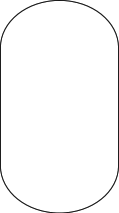This consists of two straight parts (which form the inside of the globe) and two elliptical ends (which will get folded down). The part we are most interested in is the elliptical end.

If we scale our measurements so that the inner cube has length $2$, then the parametrisation of the ellipse is:

 $\left(\mathrm{sin}\left(\varphi \right),b\mathrm{cos}\left(\varphi \right)\right),\phantom{\rule{2em}{0ex}}$

with $0. The parametrisation of the paper is therefore:

 $\left(s,\mathrm{sin}\left(\varphi \right),b\mathrm{cos}\left(\varphi \right)\right)$

To get the fold curve, we need to figure out the curve:

 $\left(b\mathrm{cos}\left(\varphi \right),{\int }_{-\pi /2}^{\varphi }\sqrt{{\mathrm{cos}}^{2}\left(\psi \right)+b{\mathrm{sin}}^{2}\left(\psi \right)}d\psi \right)$

The integral simplifies (slightly) to:

 $\begin{array}{rl}{\int }_{-\pi /2}^{\varphi }\sqrt{{\mathrm{cos}}^{2}\left(\psi \right)+{b}^{2}{\mathrm{sin}}^{2}\left(\psi \right)}d\psi & ={\int }_{0}^{\varphi }\sqrt{1-\left(1-{b}^{2}\right){\mathrm{sin}}^{2}\left(\psi \right)}d\psi \\ & ={\int }_{-\pi /2}^{\varphi }\sqrt{1-m{\mathrm{sin}}^{2}\left(\psi \right)}d\psi \end{array}$

where ${m}^{2}=1-{b}^{2}$.

This is an incomplete elliptic integral of the 2nd kind. Unless $b=1$, it has no closed form solution. Fortunately, there are numerical solutions and the scipy module for python can compute them. The program curve.py uses this to produce a table of coordinates for the paper curve which can be read in by the LaTeX document triskele.tex to produce the template; the version for $b=.8$ is triskele.pdf.

Below this is plotted with the approximation of an arc for comparison. It should be noted that they are very close.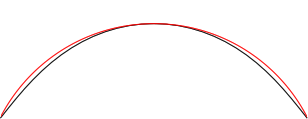If $b=1$ (whence the cross section is circular) then the integral simplifies to

 ${\int }_{-\pi /2}^{\varphi }\sqrt{{\mathrm{cos}}^{2}\left(\psi \right)+{\mathrm{sin}}^{2}\left(\psi \right)}d\psi ={\int }_{-\pi /2}^{\varphi }d\psi =\varphi +\pi /2$

and so the fold curve has equation

 $\left(\mathrm{cos}\left(\varphi \right),\varphi +\pi /2\right)$

which we can plot directly to obtain the following curve, overlaid again with an arc so show how the separation has increased.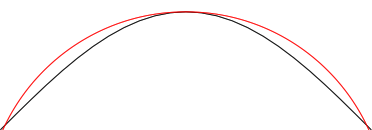Note that the case where $b=1$ means that the ends are circular and that the two folds meet at the centre.

# 5 Finding Functions

In Equation 1 there are three functions: $f\left(t\right)$, $g\left(t\right)$, and the arc length function, say $h\left(t\right)$. These are related via a Pythagorean relationship on the derivatives that states that:

$h\text{'}\left(t{\right)}^{2}=f\text{'}\left(t{\right)}^{2}+g\text{'}\left(t{\right)}^{2}$
 3

Thus the problem of finding such triples $\left(f,g,h\right)$ which have a closed form expression (for some suitable definition thereof) is closely related to a functional version of finding Pythagorean triples.

The fact that we are working with derivatives is what makes this a non-trivial question. As we have already seen, this problem is complicated even for an ellipse.

Trigonometric and hyperbolic functions form a natural source of these triples:

 $f\left(t\right)$ $g\left(t\right)$ $h\left(t\right)$ $\mathrm{sin}\left(t\right)$ $\mathrm{cos}\left(t\right)$ $t$ $\mathrm{ln}|\mathrm{cos}\left(t\right)|$ $t$ $\mathrm{ln}|\mathrm{sec}\left(t\right)+\mathrm{tan}\left(t\right)|$ $\mathrm{cosh}\left(t\right)$ $t$ $\mathrm{sinh}\left(t\right)$ ${\mathrm{tan}}^{-1}\left(\mathrm{sinh}\left(t\right)\right)$ $\mathrm{ln}\left(\mathrm{cosh}\left(t\right)\right)$ $t$ $\frac{1}{\sqrt{1+x}}$ $\frac{1}{\sqrt{1-x}}$ ${\mathrm{sin}}^{-1}\left(t\right)$